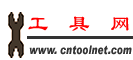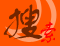工具搜索引擎 加入收藏 意见反馈 帮助中心 繁體中文 资讯标题 资讯内容 商业机会 商机简介 公司名称 公司简介 超市名称 超市简介
 首页 工具超市 产品信息 资讯中心 工具黄页 站长工具 实用工具 会员管理 联系我们

If...Then...Else 条件语句

If...Then...Else 语句

If...Then...Else语句基本结构如下：

`If ... Then`
`...`
`Else`
`...`
`End if `

`If(如果) ...(符合某些条件) Then(就)`
`  ...(执行代码)`
`Else(反之)`
`  ...(执行其它代码)`
`End if (结束)`

`Private Sub btnShow_Click(ByVal sender As System.Object, _`
`ByVal e As System.EventArgs) Handles btnShow.Click`
`    If chkSite.Checked Then`
`       lblText.Text = "You selected " & chkSite.Text`
`    Else`
`       lblText.Text = "You did not select " & chkSite.Text`
`    End If`
`End Sub`

没有 Else 的 If 语句

`If...Then`
`...`
`End if`

`Private Sub btnShow_Click(ByVal sender As System.Object, _`
`ByVal e As System.EventArgs) Handles btnShow.Click`
`    If chkSite.Checked Then`
`       lblText.Text = "You selected " & chkSite.Text`
`    End if`
` End Sub`

比较运算符 (Comparison Operators)

 比较运算符 比较运算符说明 比较运算符示例 = 等于 25 = 25  'True 26 = 25  'False > 大于 26 > 25  'True 24 > 25  'False < 小于 24 < 25  'True 26 < 25  'False <> 不等于 24 <> 25  'True 25 <> 25  'False >= 大于等于 25 >= 25  'True 26 >= 25  'True 24 >= 25  'False <= 小于等于 25 <= 25  'True 24 <= 25  'True 26 <= 25  'False

逻辑运算符 (Logical Operators) 中的 And 和 Or

`If a > b And a > c Then`

`If a > b Or a > c Then`

可执行多重选择的 ElseIf 语句

`If...Then`
`...`
`ElseIf...Then`
`...`
`Else`
`...`
`End if`

`Dim number, digits As Integer`
`number = 25`
`If number < 10 Then`
`    digits = 1`
`ElseIf number < 100 Then`
`    digits = 2`
`ElseIf number < 1000 Then`
`    digits = 3`
`Else`
`    digits = 4`
`End If`

VB 2005 入门教程

If...Then...Else 条件语句
Select...Case 条件语句
For...Next 循环语句
 暂无相关评论

 发表评论： 用户: 验证码

 版权所有 海力工具网 电话：0523-86864621